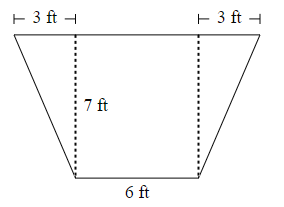ResourcesTestimonials
Plans
ResourcesTestimonials
Plans
Worksheet

# Area Of Trapezoid (Decompositon Of Areas) Worksheet

## 8 problemsIn 6th Grade geometry, the area of a trapezoid is a fundamental concept. Teachers can use  “area of trapezoid (decomposition of areas) worksheet” to help students understand this concept. Using the decomposition of areas, the trapezoid is breakdown into simpler shapes whose areas can be easily calculated. After calculating the areas of these simpler shapes students needs to add them together to find the area of the original trapezoid.

Geometry
6.G.A.1

For example: What is the area of the given trapezoid?Solution:

The given trapezoid is breakdown into 3 shapes, 1 rectangle, and 2 triangles.

For rectangle:

Length = 7 ft

Width = 6 ft

Area = Length * width = 7 * 6 = 42 ft^2

For the first triangle:

Base = 3 ft

Height = 7 ft

Area of first triangle = ½ * base * height = ½ * 3 * 7 = 10.5 ft^2

For the second triangle:

Base = 3 ft

Height = 7 ft

Area of second triangle = ½ * base * height = ½ * 3 * 7 = 10.5 ft^2

Area of the trapezoid = Area of rectangle + Area of first triangle + Area of second triangle

= 42 + 10.5 + 10.5

= 63

Therefore, the area of the trapezoid is 63 square feet.

For example: What is the area of the given trapezoid?Solution:

The given trapezoid is breakdown into 3 shapes, 1 rectangle, and 2 triangles.

For rectangle:

Length = 7 ft

Width = 6 ft

Area = Length * width = 7 * 6 = 42 ft^2

For the first triangle:

Base = 3 ft

Height = 7 ft

Area of first triangle = ½ * base * height = ½ * 3 * 7 = 10.5 ft^2

For the second triangle:

Base = 3 ft

Height = 7 ft

Area of second triangl...

Show all

### What teachers are saying about BytelearnWhat teachers are sayingStephen Abate
19-year math teacher
Carmel, CA
Any math teacher that I know would love to have access to ByteLearn.Jennifer Maschino
4-year math teacher
Summerville, SC
“I love that ByteLearn helps reduce a teacher’s workload and engages students through an interactive digital interface.”Rodolpho Loureiro
Dean, math program manager, principal
Miami, FL
“ByteLearn provides instant, customized feedback for students—a game-changer to the educational landscape.”